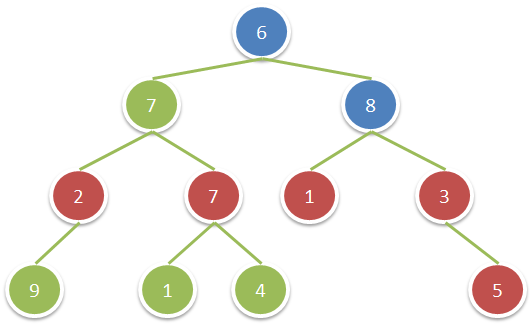### LeetCode 1313. Decompress Run-Length Encoded List

We are given a list nums of integers representing a list compressed with run-length encoding.

Consider each adjacent pair of elements [freq, val] = [nums[2*i], nums[2*i+1]] (with i >= 0). For each such pair, there are freq elements with value val concatenated in a sublist. Concatenate all the[……]

Read more

### LeetCode 1365. How Many Numbers Are Smaller Than the Current Number

Given the array nums, for each nums[i] find out how many numbers in the array are smaller than it. That is, for each nums[i] you have to count the number of valid j's such that j != i and nums[j] < nums[i].

Return the answer in an array.

Example 1:

Input: nums = [8,1,2,2,3]
Ou[……]

Read more

### LeetCode 1379. Find a Corresponding Node of a Binary Tree in a Clone of That Tree

Given two binary trees original and cloned and given a reference to a node target in the original tree.

The cloned tree is a copy of the original tree.

Return a reference to the same node in the cloned tree.

Note that you are not allowed to change any of the two trees or the target node and[……]

Read more

### LeetCode 1614. Maximum Nesting Depth of the Parentheses

A string is a valid parentheses string (denoted VPS) if it meets one of the following:

It is an empty string "", or a single character not equal to "(" or ")",
It can be written as AB (A concatenated with B), where A and B are VPS's, or
It can be written as (A[……]

Read more

### LeetCode 1221. Split a String in Balanced Strings

Balanced strings are those who have equal quantity of 'L' and 'R' characters.

Given a balanced string s split it in the maximum amount of balanced strings.

Return the maximum amount of splitted balanced strings.

Example 1:

Input: s = "RLRRLLRLRL"
Outpu[……]

Read more

### LeetCode 1315. Sum of Nodes with Even-Valued Grandparent

Given a binary tree, return the sum of values of nodes with even-valued grandparent. (A grandparent of a node is the parent of its parent, if it exists.)

If there are no nodes with an even-valued grandparent, return 0.

Example 1:Input: root = [6,7,8,2,7,1,3,9,null,1,4,null,null,n[……]

Read more

### LeetCode 1395. Count Number of Teams

There are n soldiers standing in a line. Each soldier is assigned a unique rating value.

You have to form a team of 3 soldiers amongst them under the following rules:

Choose 3 soldiers with index (i, j, k) with rating (rating[i], rating[j], rating[k]).
A team is valid if: (rating[i] < rat[……]

Read more

### LeetCode 1282. Group the People Given the Group Size They Belong To

There are n people that are split into some unknown number of groups. Each person is labeled with a unique ID from 0 to n – 1.

You are given an integer array groupSizes, where groupSizes[i] is the size of the group that person i is in. For example, if groupSizes = 3, then person 1 must be in a[……]

Read more

### LeetCode 1528. Shuffle String

Given a string s and an integer array indices of the same length.

The string s will be shuffled such that the character at the ith position moves to indices[i] in the shuffled string.

Return the shuffled string.

Example 1:Input: s = "codeleet", indices = [4,5,6,7,0,2,[……]

Read more

### LeetCode 1342. Number of Steps to Reduce a Number to Zero

Given a non-negative integer num, return the number of steps to reduce it to zero. If the current number is even, you have to divide it by 2, otherwise, you have to subtract 1 from it.

Example 1:

Input: num = 14
Output: 6
Explanation:
Step 1) 14 is even; divide by 2 and obtain 7.
St[……]

Read more